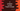# C program to print Floyd's triangle# Introduction :

In this tutorial, we will learn how to print Floyd’s triangle in C programming language. It looks like as below :

``````1
2 3
4 5 6
7 8 9 10
11 12 13 14 15 ``````

This is Floyd’s triangle of height 5.

Our program will ask the user to enter the height of the triangle. It will print that triangle on the console based on the height. I will show you two different ways to print it.

### Method 1: Using two loops :

We can print this triangle using two for loops. The outer loop will run for height number of times i.e. if the height of the triangle is 5, it will run for 5 times. The inner loop will run to print the numbers of the triangle. It will run for the current value of the outer loop times. We will keep one variable to print the values of the triangle. This variable will increase by 1 on each step.

``````#include <stdio.h>

int main()
{
int rowCount;
int currentRow;
int currentColumn;
int currentValue = 1;

printf("Enter total number of rows :");
scanf("%d", &rowCount);

for (currentRow = 1; currentRow <= rowCount; currentRow++)
{
for (currentColumn = 1; currentColumn <= currentRow; currentColumn++)
{
printf("%d ", currentValue);
currentValue++;
}
printf("\n");
}
}``````

### Sample Output :

``````Enter total number of rows :5
1
2 3
4 5 6
7 8 9 10
11 12 13 14 15 ``````

### Method 2: Recursive approach :

We can also print Floyd’s triangle recursively. It will call the same function recursively to print the rows :

``````#include <stdio.h>

void printFloyd(int height, int currentRow, int currentValue)
{
if (height < currentRow)
{
return;
}
int i = 1;

for (i = 1; i <= currentRow; i++)
{
printf("%d ", currentValue);
currentValue++;
}

printf("\n");
return printFloyd(height, currentRow + 1, currentValue);
}

int main()
{
int rowCount;

printf("Enter total number of rows :");
scanf("%d", &rowCount);

printFloyd(rowCount, 1, 1);
}``````

### Sample Output :

``````Enter total number of rows :5
1
2 3
4 5 6
7 8 9 10
11 12 13 14 15 ``````

### Where is the color and why codevscolor ?

Long story short, I love paintings and I paint on weekends. We(me and my wife) have one Youtube channel. Don't forget to subscribe ❤️❤️🤩